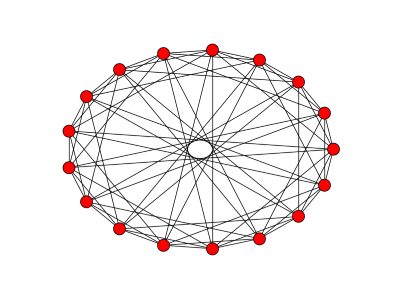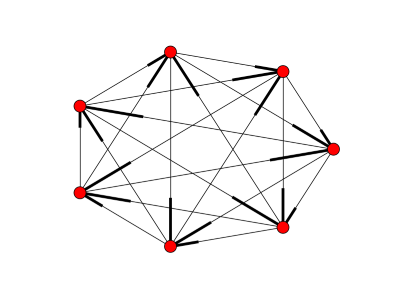# Graphs and square roots modulo a prime

Imagine a clock with a prime number of hours. So instead of being divided into 12 hours, it might be divided into 11 or 13, for example. You can add numbers on this clock the way you’d add numbers on an ordinary clock: add the two numbers as ordinary integers and take the remainder by p, the number of hours on the clock. You can multiply them the same way: multiply as ordinary integers, then take the remainder by p. This is called arithmetic modulo p, or just mod p.

Which numbers have square roots mod p? That is, for which numbers x does there exist a number y such that y2 = x mod p? It turns out that of the non-zero numbers, half have a square root and half don’t. The numbers that have square roots are called quadratic residues and the ones that do not have square roots are called quadratic nonresidues. Zero is usually left out of the conversation. For example, if you square the numbers 1 through 6 and take the remainder mod 7, you get 1, 4, 2, 2, 4, and 1. So mod 7 the quadratic residues are 1, 2, and 4, and the nonresidues are 3, 5, and 6.

A simple, brute force way to tell whether a number is a quadratic residue mod p is to square all the numbers from 1 to p-1 and see if any of the results equals your number. There are more efficient algorithms, but this is the easiest to understand.

At first it seems that the quadratic residues and nonresidues are scattered randomly. For example, here are the quadratic residues mod 23:

[1, 2, 3, 4, 6, 8, 9, 12, 13, 16, 18]

But there are patterns, such as the famous quadratic reciprocity theorem. We can see some pattern in the residues by visualizing them on a graph. We make the numbers mod p vertices of a graph, and join two vertices a and b with an edge if ab is a quadratic residue mod p.

Here’s the resulting graph mod 13:And here’s the corresponding graph for p = 17:And here’s Python code to produce these graphs:

```import networkx as nx
import matplotlib.pyplot as plt

def residue(x, p):
for i in range(1, p):
if (i*i - x) % p == 0:
return True
return False

p = 17
G = nx.Graph()
for i in range(p):
for j in range(i+1, p):
if residue(i-j, p):

nx.draw_circular(G)
plt.show()
```

However, this isn’t the appropriate graph for all values of p. The graphs above are undirected graphs. We’ve implicitly assumed that if there’s an edge between a and b then there should be an edge between b and a. And that’s true for p = 13 or 17, but not, for example, for p = 11. Why’s that?

If p leaves a remainder of 1 when divided by 4, i.e. p = 1 mod 4, then x is a quadratic residue mod p if and only if –x is a quadratic residue mod p. So if ab is a residue, so is ba. This means an undirected graph is appropriate. But if p = 3 mod 4, x is a residue mod p if and only if –x is a non-residue mod p. This means we should use directed graphs if p = 3 mod 4. Here’s an example for p = 7.The code for creating the directed graphs is a little different:

```G = nx.DiGraph()
for i in range(p):
for j in range(p):
if residue(i-j, p):
```

Unfortunately, the way NetworkX draws directed graphs is disappointing. A directed edge from a to b is drawn with a heavy line segment on the b end. Any suggestions for a Python package that draws more attractive directed graphs?

The idea of creating graphs this way came from Roger Cook’s chapter on number theory applications in Graph Connections. (I’m not related to Roger Cook as far as I know.)

You could look at quadratic residues modulo composite numbers too. And I imagine you could also make some interesting graphs using Legendre symbols.

## 5 thoughts on “Graphs and square roots modulo a prime”

1. Random Internet Stranger

The only thing I have ever found that draws nice looking graphs in a reliable way is pydot, pygraphviz, etc. Of course these rely on graphviz being installed, and they create a subprocess to do the work, so it is not ideal.

2. Thanks. The NetworkX documentation says it’s too hard to get the arrows to work using the matplotlib library it is built on. So it makes sense that something with different basic graphic routines, like graphviz, might handle arrows better. Aside from directed graphs, I find the asthetics/effort ratio to be higher with NetworkX.

3. Kevin Coombes

What do you do in the composite case when there are nonzero square roots of zero (like 3 mod 9)? Do you still ignore them? Or do you then connect each node to itself?

Also, what happens with cubic residues? I suppose the natural generalization to that question would look at qth residues mod p, where q is prime. Even more generally, mth residues mod n where m and n are relatively prime.

4. Kevin: I suppose the analogous thing to do would be to ignore square roots of zero. That’s sorta what the Legendre symbol does.

Your cubic question made me think it might be interesting to associate not just edges but faces etc. with the integers mod p (or mod a composite) and create something where the homology tells you something, or at least illustrates something you already know.

5. Guy Musch

Modulo a composite number, the product of two residues is a residue. The product of a residue and a nonresidue may be a residue, a nonresidue, or zero.